Electron. J. Differential Equations, Vol. 2020 (2020), No. 30, pp. 1-12.

### S-asymptotically omega-periodic mild solutions to fractional differential equations Darin Brindle, Gaston M. N'Guerekata

Abstract:
This article concerns the existence of mild solutions to the semilinear fractional differential equation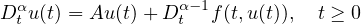with nonlocal conditions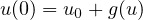where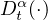(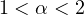) is the Riemann-Liouville derivative,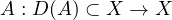is a linear densely defined operator of sectorial type on a complex Banach space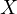,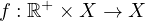is S-asymptotically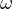-periodic with respect to the first variable. We use the Krsnoselskii's theorem to prove our main theorem. The results obtained are new even in the context of asymptotically-periodic functions. An application to fractional relaxation-oscillation equations is given.

Submitted August 11, 2019. Published April 7, 2020.
Math Subject Classifications: 34G20, 34G10.
Key Words: S-asymptotically omega-periodic sequence; fractional semilinear differential equation.

An addendum was posted on April 18, 2020. It corrects Theorem 2.9 and its proof. See the last page of this article.

Show me the PDF file (358 KB), TEX file for this article.Darin Brindle Department of Mathematics Morgan State University Baltimore, MD 21251, USA email: Darin.Brindle@morgan.edu Gaston M. N'Guérékata Department of Mathematics Morgan State University Baltimore, MD 21251, USA email: Gaston.N'Guerekata@morgan.edu